http://michaelpollmeier.com
https://github.com/mpollmeier/gremlin-scala/

### Graph theory started in the 18th century• a set of vertices
• a set of edges between vertices

### Graph in computer science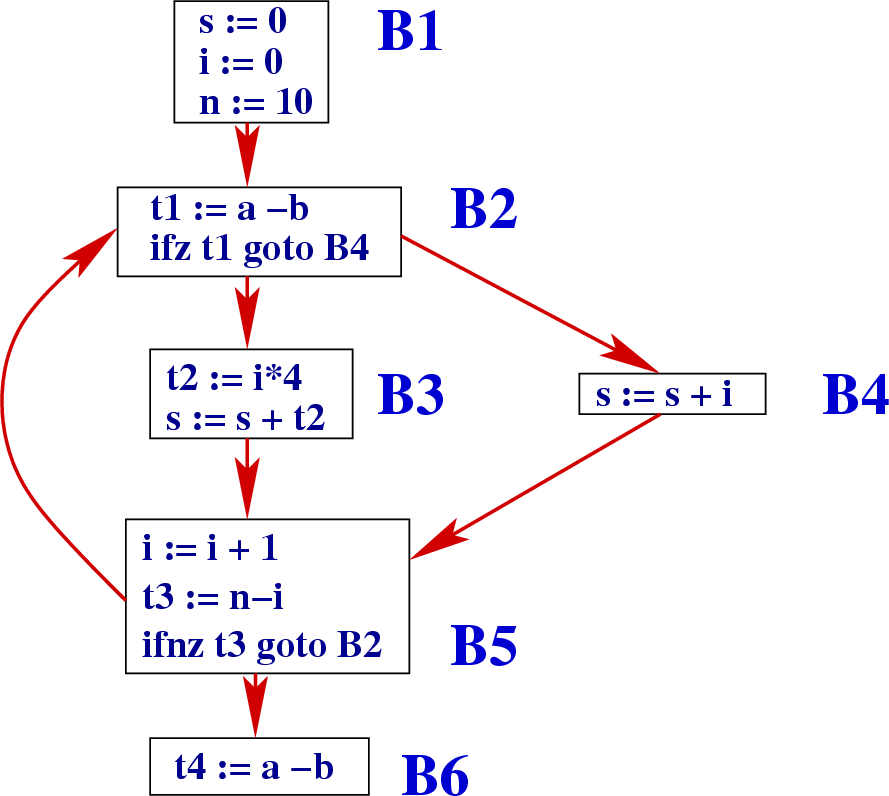compiler optimizations

ensime

### Graph in Web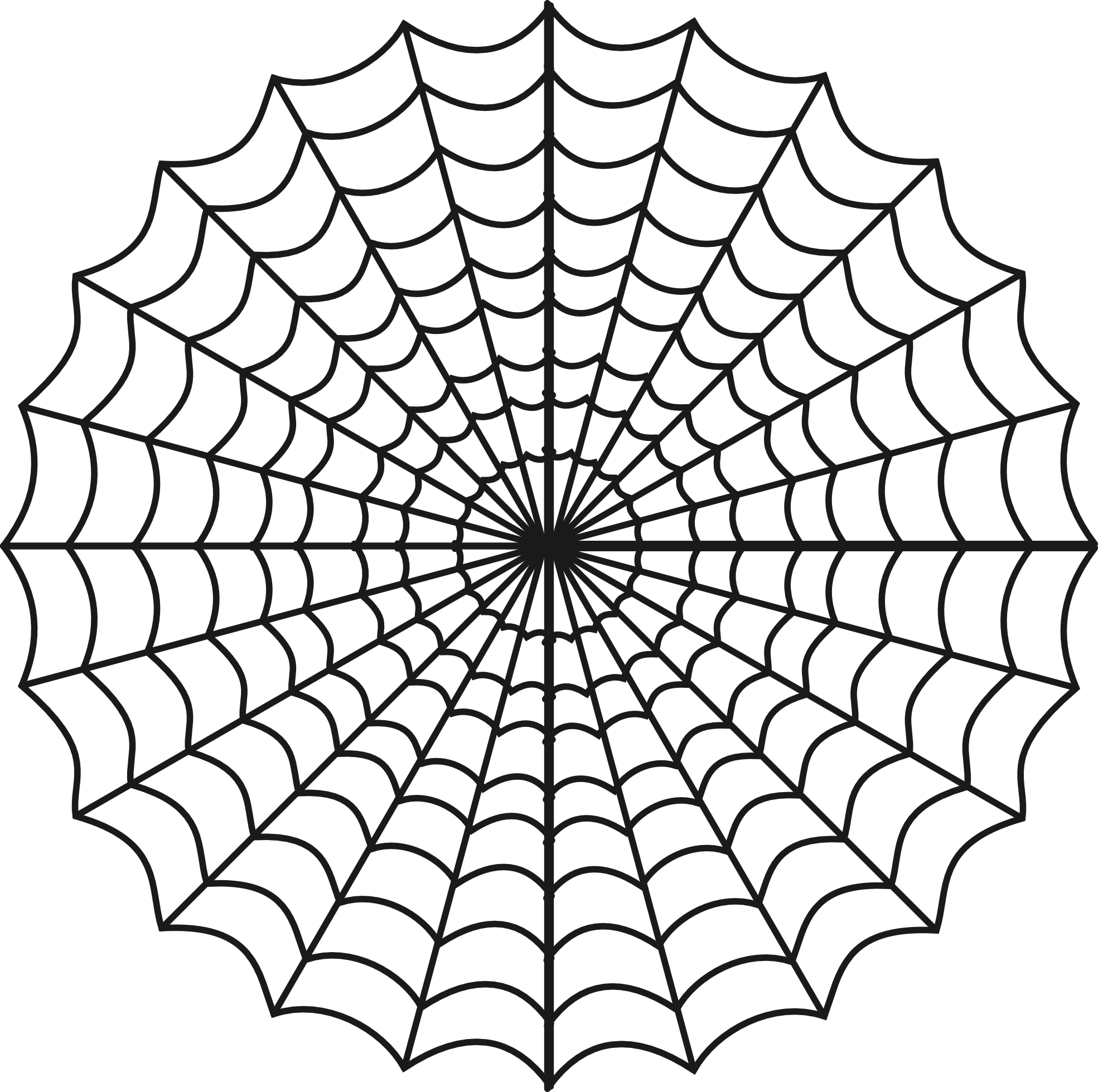the WWW (hypertexts)

### Engineeringpipe network analysis

### Electrical engineeringcircuit theory

### Linguistics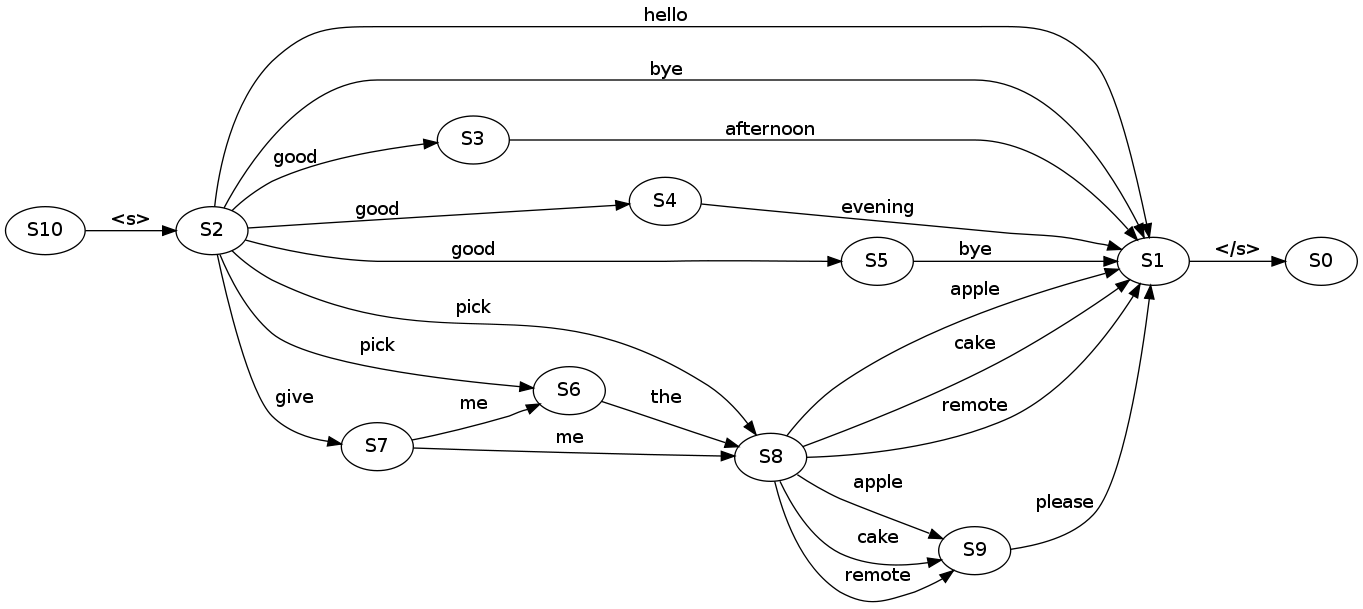language models

### Biology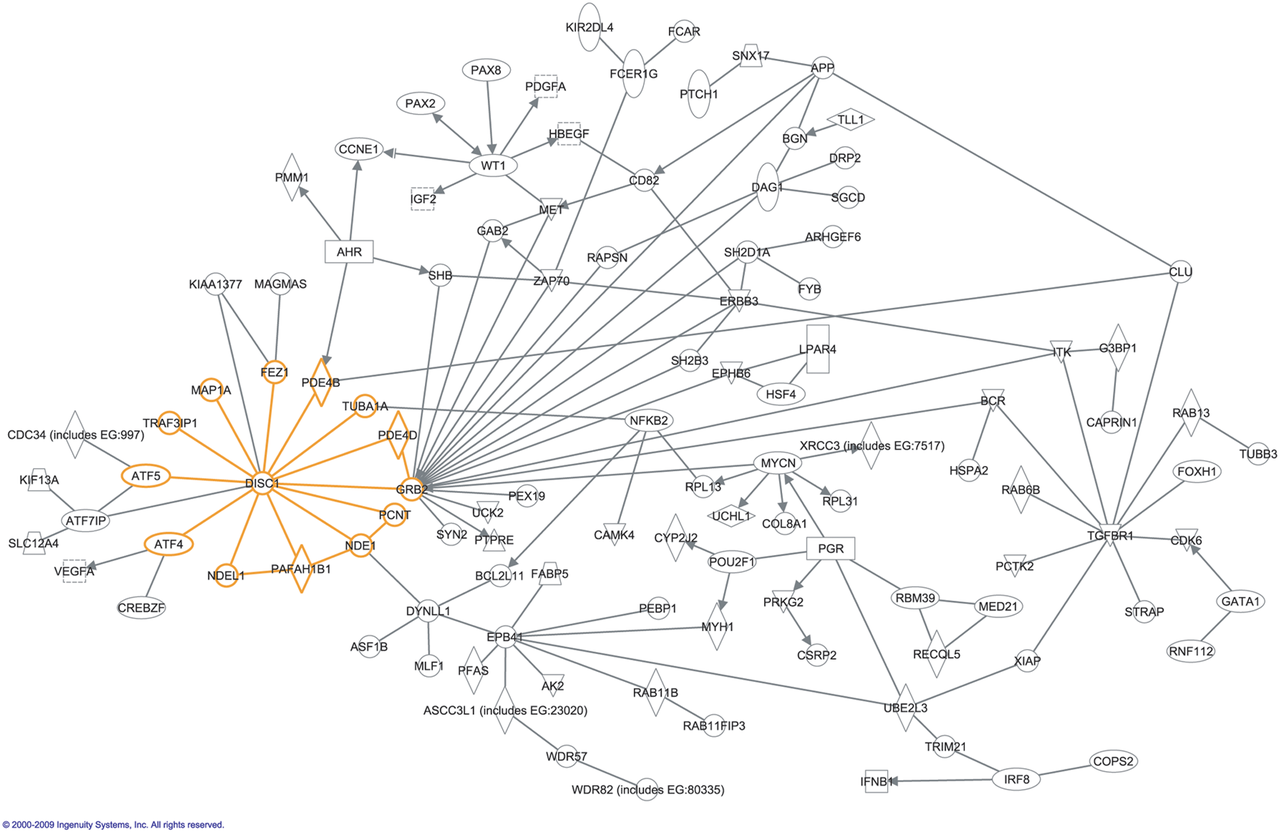gene interactions

### Social Networks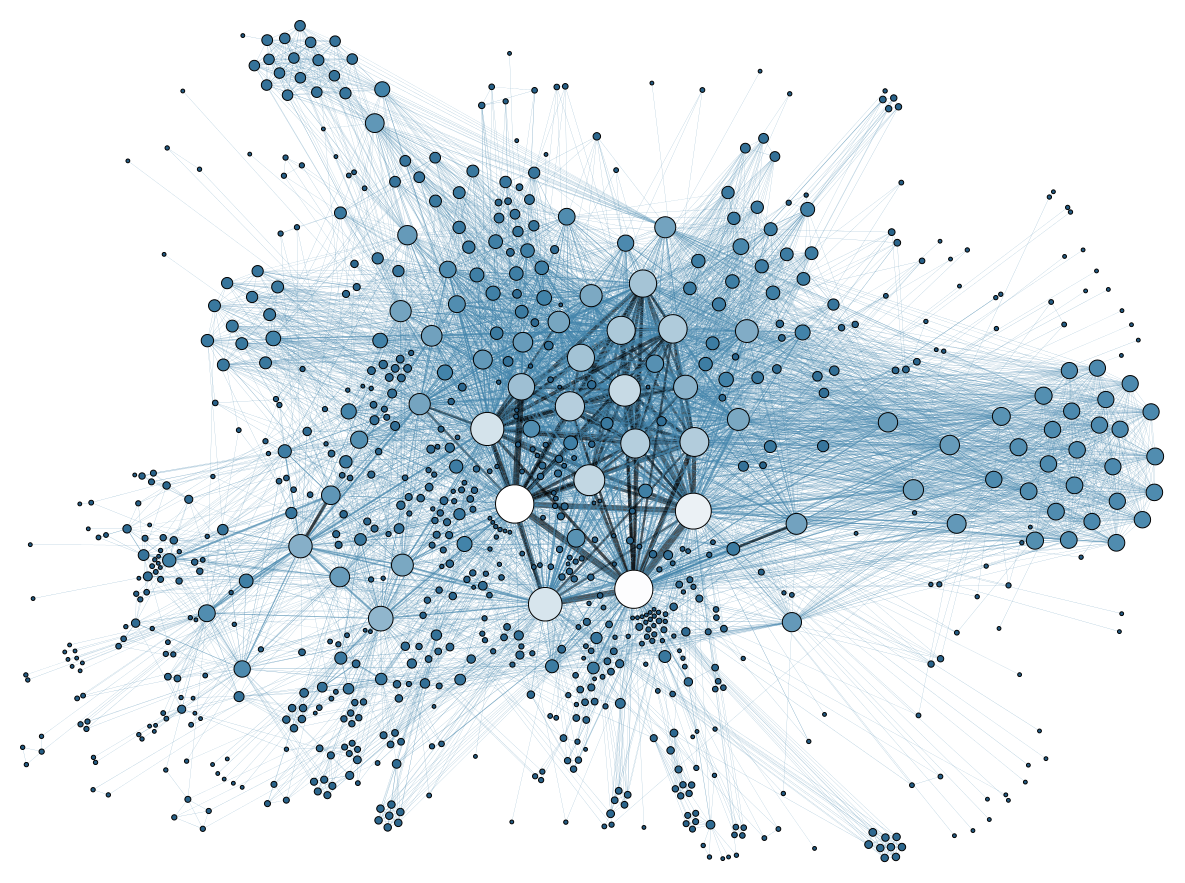### Relational model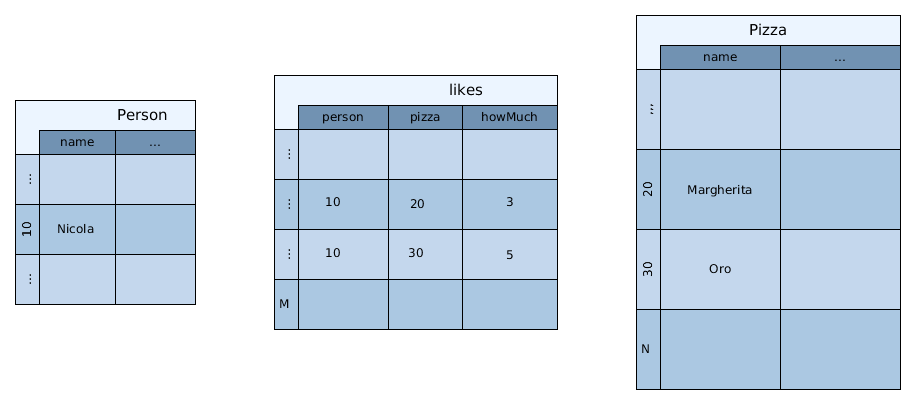### Graph model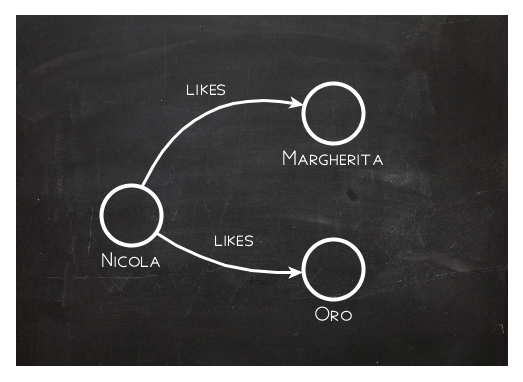just as you would draw it

### RDB: find pizzas liked by Nicola

• given a Person (Nicola)
• find Nicola (id=10) in likes table: O(log N)
• find 'Margherita' and 'Oro' in Pizza table: O(log N)
``````
SELECT pizza.name
FROM
Person as person
JOIN Likes as likes ON person.id = likes.person
JOIN Pizza as pizza ON likes.pizza = pizza.id AND
WHERE person.id = 10
``````

### Gremlin: find pizzas liked by Nicola

• given a Person (Nicola)
• follow all likes edges: O(1)
``````
graph.V(10).out("likes")
``````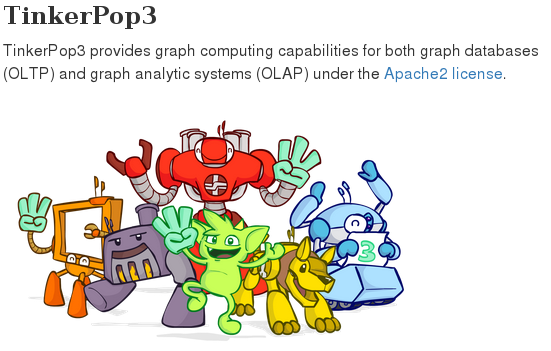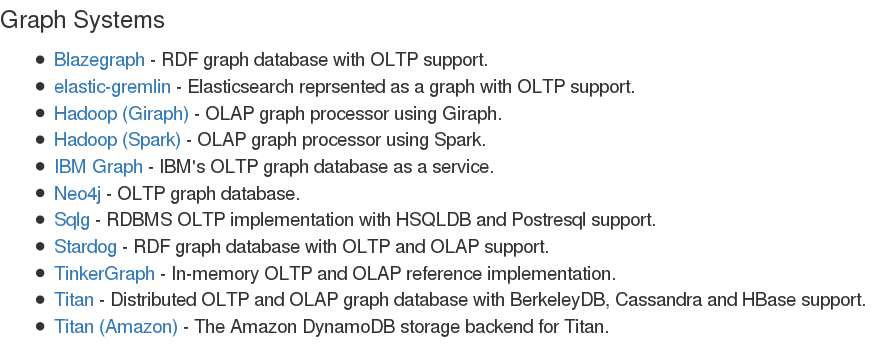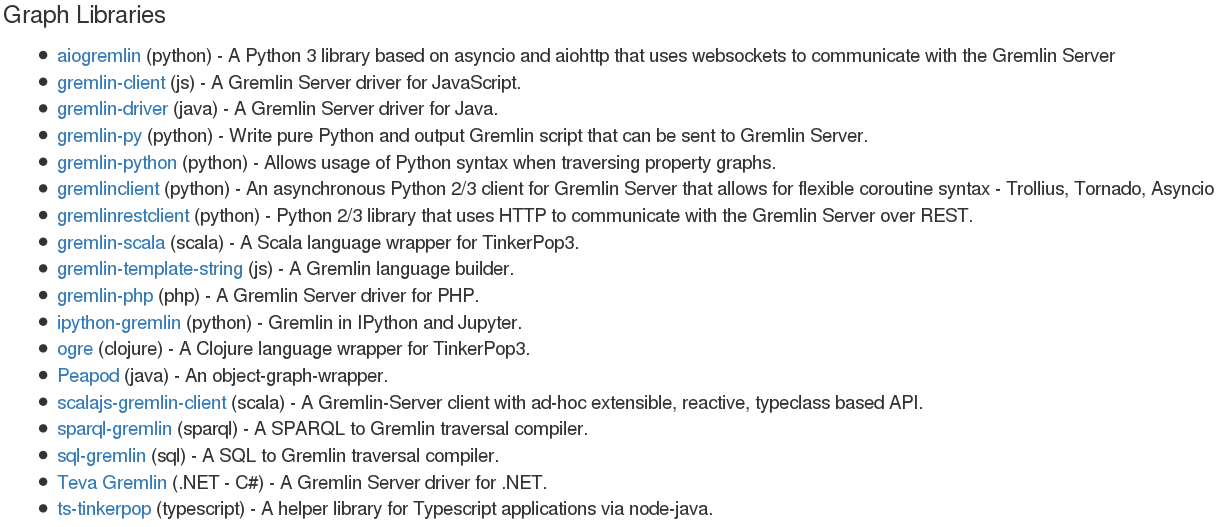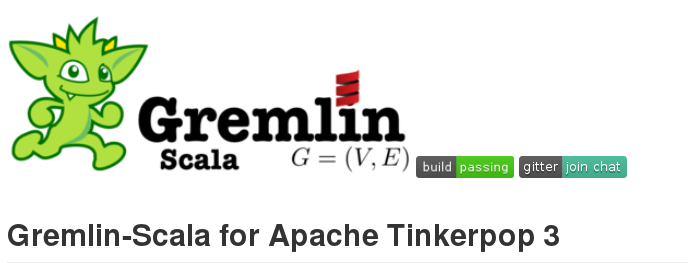https://github.com/mpollmeier/gremlin-scala

### Create vertices and edges

``````
val graph = TinkerGraph.open.asScala

// typed keys
val Founded = Key[String]("founded")
val Distance = Key[Int]("distance")

// two vertices
val paris = graph + "Paris"
val london = graph + ("London", Founded → "43 AD")

// some edges
paris <-- "OtherWayAround" --- london
paris <-- "Eurostar" --> london
paris --- ("Eurostar", Distance → 495) --> london
``````

### Compile time safety #1

``````
``````

### Compile time safety #2

``````
graph.V.outE.inV  //compiles
graph.V.outE.outE //does _not_ compile
``````

### Compile time safety #3

``````
paris.as("x")
.outE("Eurostar").as("y")
.value(Distance).as("z")
.select
// returns `(Vertex, Edge, Int)` for each path
``````

### For comprehensions

``````
for {
person   <- graph.V.hasLabel("person")
favorite <- person.outE("likes")
.orderBy("weight", Order.decr)
.limit(1)
.inV
} yield (person, favorite.label)
// returns (Vertex, String)
``````

### Mapping vertices from/to case classes

``````
@label("example")
case class Example(@id id: Option[Int],
longValue: Long,
stringValue: Option[String])

val example = Example(None, Long.MaxValue, Some("optional value"))
val v: Vertex = graph + example
v.toCC[Example] // equal to `example`, but with id set

graph.V.hasLabel[Example]
``````### Performance depends on db and architecture

• connection to db: remote, local or in memory?
• minimise need to serialise data over the wire
• usage of indexes
• DB driver maturity

### big data apps: depending on the driver and use case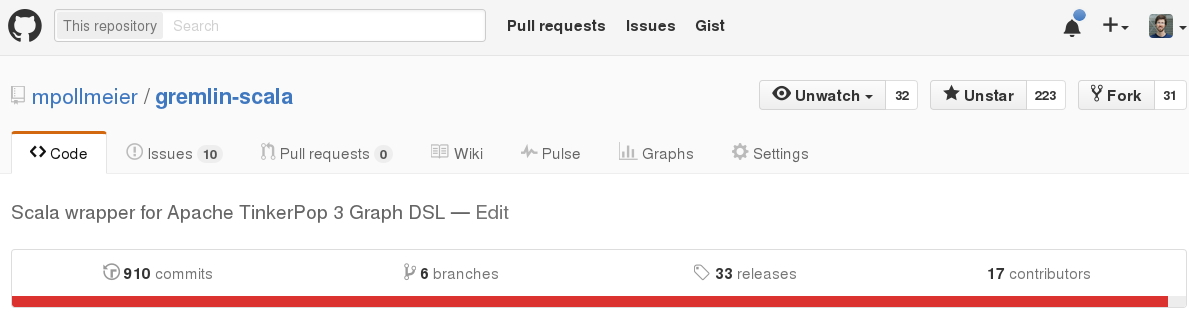https://github.com/mpollmeier/gremlin-scala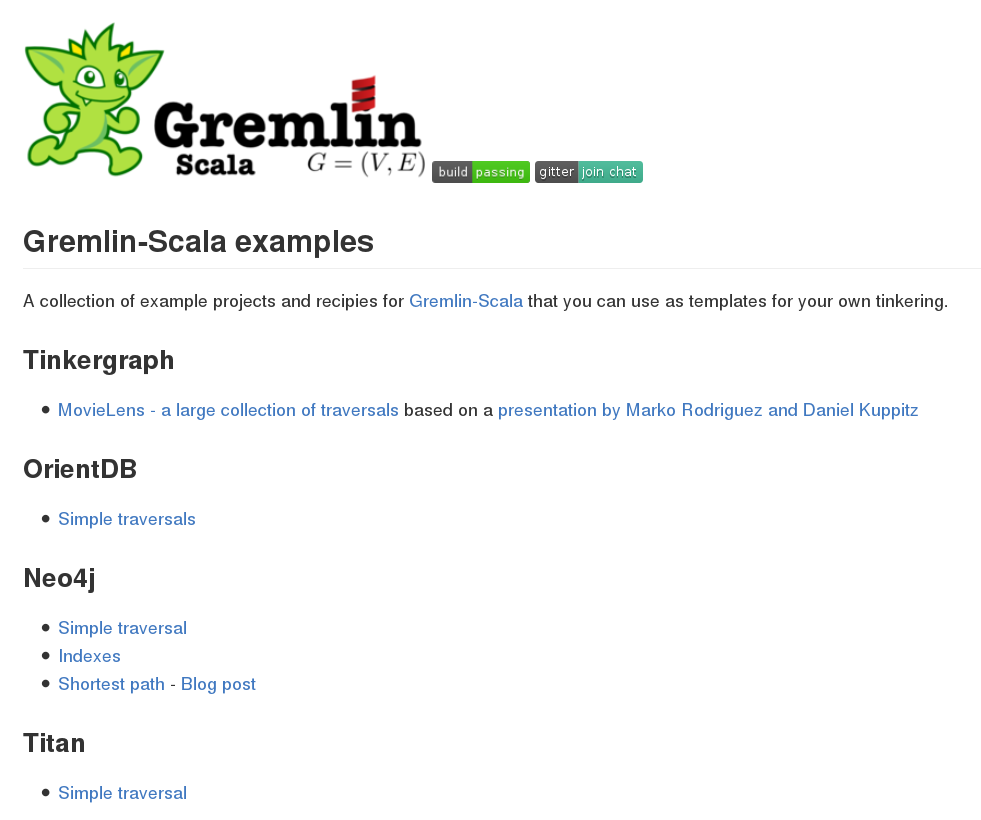https://github.com/mpollmeier/gremlin-scala-examples

### Questions?kudos to ryanmart - I stole some of his slides